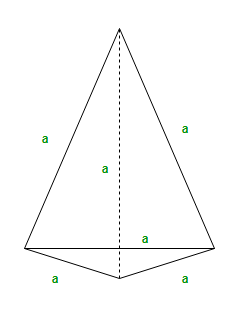# Program to calculate area and volume of a Tetrahedron

• Difficulty Level : Basic
• Last Updated : 07 Aug, 2022

Time complexity: O(1)

space complexity: O(1)A Tetrahedron is simply a pyramid with a triangular base. It is a solid object with four triangular faces, three on the sides or lateral faces, one on the bottom or the base and four vertices or corners. If the faces are all congruent equilateral triangles, then the tetrahedron is called regular.The area of Tetrahedron can be found by using the formula :

`Area = sqrt(3)*(side*side)`

Examples :

```Input : side = 3
Output : 15.5885

Input : side = 20
Output : 692.82```

## C++

 `// C++ Program to Calculate``// area of tetrahedron``#include``#include``using` `namespace` `std;`` ` `//Utility Function``double` `area_of_tetrahedron(``int` `side)``{``    ``return` `(``sqrt``(3)*(side*side));``}` `//Driver Code``int` `main()``{``    ``int` `side=3;``    ``cout<< ``"Area of Tetrahedron ="``        ``<< area_of_tetrahedron(side);``}` `// This code is contributed by anant321.`

## Java

 `// Java Program to Calculate``// area of tetrahedron``import` `java.util.*;``import` `java.lang.*;` `class` `GFG {` `    ``// Utility Function``    ``public` `static` `double` `area_of_tetrahedron(``int` `side)``    ``{``        ``return` `(Math.sqrt(``3``) * (side * side));` `    ``}` `    ``// Driver code``    ``public` `static` `void` `main(String[] args)``    ``{``        ``int` `side = ``3``;``        ``System.out.println(``"Area of Tetrahedron ="``                     ``+ area_of_tetrahedron(side));``    ``}``}``// This code is contributed``// by Prasad Kshirsagar`

## Python3

 `# Python3 Program to``# Calculate area of``# tetrahedron``import` `math` `def` `area_of_tetrahedron(side):``    ``return` `(math.sqrt(``3``) ``*``           ``(side ``*` `side));` `# Driver Code``side ``=` `3``;``print``(``"Area of Tetrahedron = "``,``       ``round``(area_of_tetrahedron(side), ``4``));` `# This code is contributed by mits`

## C#

 `// C# Program to Calculate``// area of tetrahedron``using` `System;` `class` `GFG``{``// Utility Function``public` `static` `double` `area_of_tetrahedron(``int` `side)``{``    ``return` `(Math.Sqrt(3) *``           ``(side * side));` `}` `// Driver code``static` `public` `void` `Main ()``{``    ``int` `side = 3;``    ``Console.WriteLine(``"Area of Tetrahedron = "` `+``                     ``area_of_tetrahedron(side));``}``}` `// This code is contributed``// by akt_mit`

## PHP

 ``

## Javascript

 `

Output :

`Area of Tetrahedron =15.5885`

The volume of the tetrahedron can be found by using the following formula :

Volume = a3/(6√2)

Examples :

```Input : side = 3
Output : 3.18

Input : side = 20
Output : 942.81```

## C++

 `// C++ code to find the volume of a tetrahedron``#include ``using` `namespace` `std;` `// Function to calculate volume``double` `vol_tetra(``int` `side)``{``    ``double` `volume = (``pow``(side, 3) /``                    ``(6 * ``sqrt``(2)));``    ``return` `volume;``}` `// Driver code``int` `main()``{``    ``int` `side = 3;``    ``double` `vol = vol_tetra(side);``    ``vol = (``double``)round(vol * 100) / 100;``    ``cout << vol;` `    ``return` `0;``}` `// This code is contributed by suresh07`

## Java

 `// Java code to find the volume of a tetrahedron``import` `java.io.*;` `class` `Tetrahedron {``    ``// Function to calculate volume``    ``static` `double` `vol_tetra(``int` `side)``    ``{``        ``double` `volume = (Math.pow(side, ``3``) / (``6` `* Math.sqrt(``2``)));``        ``return` `volume;``    ``}` `    ``// Driver Code``    ``public` `static` `void` `main(String[] args)``    ``{``        ``int` `side = ``3``;``        ``double` `vol = vol_tetra(side);``        ``vol = (``double``)Math.round(vol * ``100``) / ``100``;``        ``System.out.println(vol);``    ``}``}`

## Python3

 `# Python code to find the volume of a tetrahedron``import` `math``def` `vol_tetra(side):``    ``volume ``=` `(side ``*``*` `3` `/` `(``6` `*` `math.sqrt(``2``)))``    ``return` `round``(volume, ``2``)` `# Driver Code``side ``=` `3``vol ``=` `vol_tetra(side)``print``(vol)`

## C#

 `// C# code to find the volume of a tetrahedron``using` `System;` `class` `Tetrahedron {``    ``// Function to calculate volume``    ``static` `double` `vol_tetra(``int` `side)``    ``{``        ``double` `volume = (Math.Pow(side, 3) / (6 * Math.Sqrt(2)));``        ``return` `volume;``    ``}` `    ``// Driver Code``    ``public` `static` `void` `Main()``    ``{``        ``int` `side = 3;``        ``double` `vol = vol_tetra(side);``        ``vol = (``double``)Math.Round(vol * 100) / 100;``        ``Console.WriteLine(vol);``    ``}``}` `// This code is contributed``// by vt_m.`

## PHP

 ``

## Javascript

 ``

Output :

`3.18`

Time complexity: O(1)
Auxiliary Space: O(1)

My Personal Notes arrow_drop_up